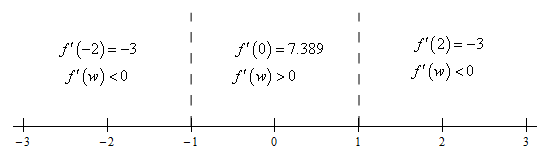Paul's Online Notes
Home / Calculus I / Applications of Derivatives / The Shape of a Graph, Part I
Show Mobile Notice Show All Notes Hide All Notes
Mobile Notice
You appear to be on a device with a "narrow" screen width (i.e. you are probably on a mobile phone). Due to the nature of the mathematics on this site it is best views in landscape mode. If your device is not in landscape mode many of the equations will run off the side of your device (should be able to scroll to see them) and some of the menu items will be cut off due to the narrow screen width.

### Section 4.5 : The Shape of a Graph, Part I

11. For $$f\left( w \right) = w{{\bf{e}}^{2\, - \,\,{\frac{1}{2}}{w^{\,2}}}}$$ answer each of the following questions.

1. Identify the critical points of the function.
2. Determine the intervals on which the function increases and decreases.
3. Classify the critical points as relative maximums, relative minimums or neither.

Show All Solutions Hide All Solutions

a Identify the critical points of the function. Show Solution

We need the 1st derivative to get the critical points so here it is.

$f'\left( w \right) = {{\bf{e}}^{2\, - \,\,{\frac{1}{2}}{w^{\,2}}}} - {w^2}{{\bf{e}}^{2\, - \,\,{\frac{1}{2}}{w^{\,2}}}} = {{\bf{e}}^{2\, - \,\,{\frac{1}{2}}{w^{\,2}}}}\left( {1 - {w^2}} \right) = {{\bf{e}}^{2\, - \,\,{\frac{1}{2}}{w^{\,2}}}}\left( {1 - w} \right)\left( {1 + w} \right)$

Now, recall that critical points are where the derivative doesn’t exist or is zero. Because we simplified and factored the derivative as much as possible we can clearly see that the derivative will exist everywhere (it’s the product of functions that exist everywhere). We can also easily see where the derivative is zero. The critical points of this function are then,

$\require{bbox} \bbox[2pt,border:1px solid black]{{w = - 1,\,\,\,\,\,\,w = 1}}$

b Determine the intervals on which the function increases and decreases. Show Solution

To determine the increase/decrease information for the function all we need is a quick number line for the derivative. Here is the number line.From this we get the following increasing/decreasing information for the function.

$\require{bbox} \bbox[2pt,border:1px solid black]{{{\mbox{Increasing : }}\left( { - 1,1} \right)\hspace{0.25in}\hspace{0.25in}{\mbox{Decreasing : }}\,\,\left( { - \infty , - 1} \right)\,\,\,\,\& \,\,\,\,\left( {1,\infty } \right)}}$

c Classify the critical points as relative maximums, relative minimums or neither. Show Solution

With the increasing/decreasing information from the previous step we can easily classify the critical points using the 1st derivative test. Here is classification of the functions critical points.

\require{bbox} \bbox[2pt,border:1px solid black]{\begin{align*}w & = - 1\,\,\,\,\,\,:\,{\mbox{ Relative Minimum}}\\ w & = 1\,\,\,\,\,\,\,\,\,:\,{\mbox{ Relative Maximum}}\end{align*}}